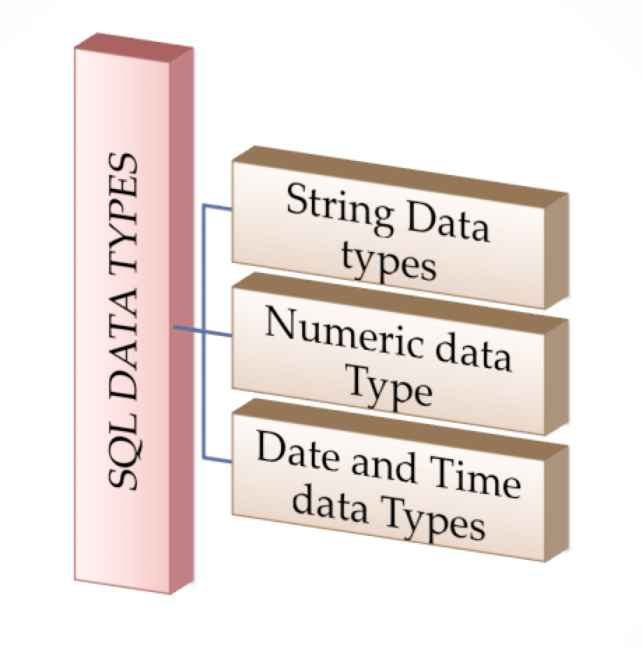Tutorials Hut

• # SQL DataTypes : String , Numeric and DateTime

Below are the frequently used Sql dataTypes in sql testing

Data types mainly classified into three categories

• String Data types
• Numeric Data types
• Date and time Data types## String SQL DataTypes:

#### Description

CHAR(size)

Used for a fixed length string

It can contain letters, numbers, and special characters.

The size can be from 0 to 255.

Default is 1

VARCHAR(size)

Used to specify a variable length string that can contain numbers, letters, and special characters

The size  can be from 0 to 65535

BINARY(size)

It is equal to CHAR(), but stores binary byte strings. The size parameter states the column length in bytes.

Default is 1

VARBINARY(size)It is equal to VARCHAR(), but it stores binary byte strings. The size parameter states the maximum column length in bytes.
TEXT(size)Holds a string which contains a maximum length of 65,535 bytes
LONGTEXTHolds a string with a maximum length of 4,294,967,295 characters
ENUM(val1, val2, …)There is no type called ENUM, an ENUM is a string object with a value chosen  from a list of permitted values , can list 65535 values in an ENUM list.
SET(val1, val2, val3, …)A string that can have 0 or more values, chosen from a list of possible values, can list up to 64 values in a SET list

## Numeric Data Types

#### Description

BIT(size)

Can take 0, 1, or NULL values.

The size parameter can hold a value from 1 to 64.

The default value for size is 1.

TINYINT(size)

It is used for a very small integer

The range is from 0 to 255.

BOOL

Zero is considered as false

Nonzero values are considered as true.

BOOLEANSame as BOOL
SMALLINT

Used for a small integer.

Range is from -32768 to 32767

MEDIUMINT

Used for a medium integer.

Range is from -8388608 to 8388607.

INT(size)

Used to specify a medium integer.

Range is from -2147483648 to 2147483647.

INTEGER(size)Equal to INT(size)
BIGINT(size)

A large integer.

Range is from -9223372036854775808 to 9223372036854775807.

FLOAT(p)

A floating point number.

P = used to determine whether to use FLOAT or DOUBLE

If p is from 0 to 24, the data type becomes FLOAT().

If p is from 25 to 53, the data type becomes DOUBLE()

DOUBLE(sized)

A normal-size floating point number.

size =The total number of digits

d= The number of digits after the decimal point

DECIMAL(sized)

It is used to specify a exact fixed-point number.

size =The total number of digits (maximum is 65)

d= The number of digits after the decimal point (maximum is 30)

DEC(sized)Equal to DECIMAL(size,d)

## Date and Time Data Types

#### Description

DATE

A date. Format: YYYY-MM-DD.

Range is from ‘1000-01-01’ to ‘9999-12-31’

DATETIME(fsp)

A date and time combination.

Format: YYYY-MM-DD hh:mm:ss.

Range is from ‘1000-01-01 00:00:00’ to ‘9999-12-31 23:59:59’.

TIMESTAMP(fsp)

Used to specify a timestamp.

Its format is YYYY-MM-DD hh:mm:ss

Range is from ‘1970-01-01 00:00:01’ UTC to ‘2038-01-09 03:14:07’ UTC.

TIME(fsp)

Used to specify a time.

Its format is hh:mm:ss.

The range is from ‘-838:59:59’ to ‘838:59:59’

YEAR

Used to specify a year in four-digit format.

Values allowed in four-digit format: 1901 to 2155, and 0000.

Recommended Articles: